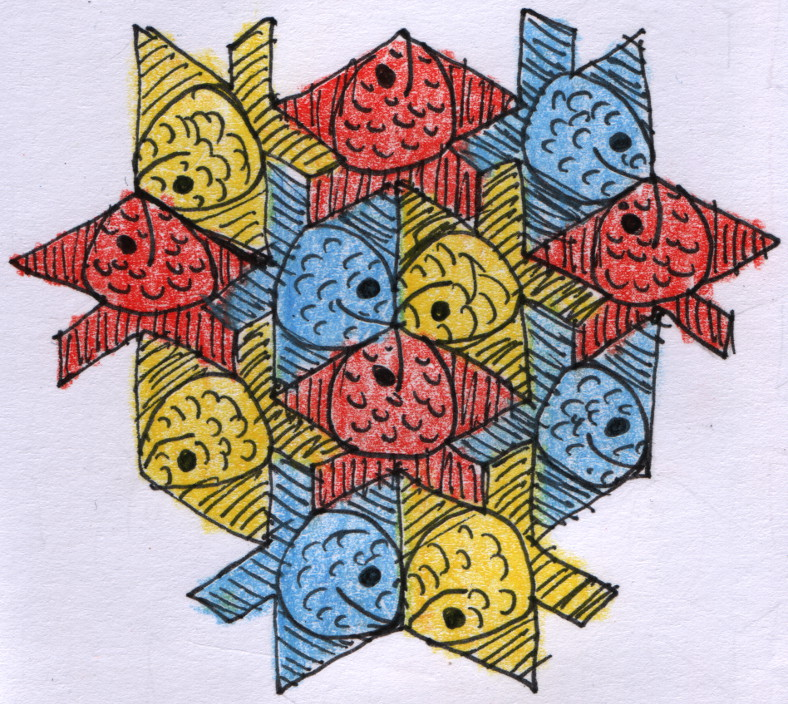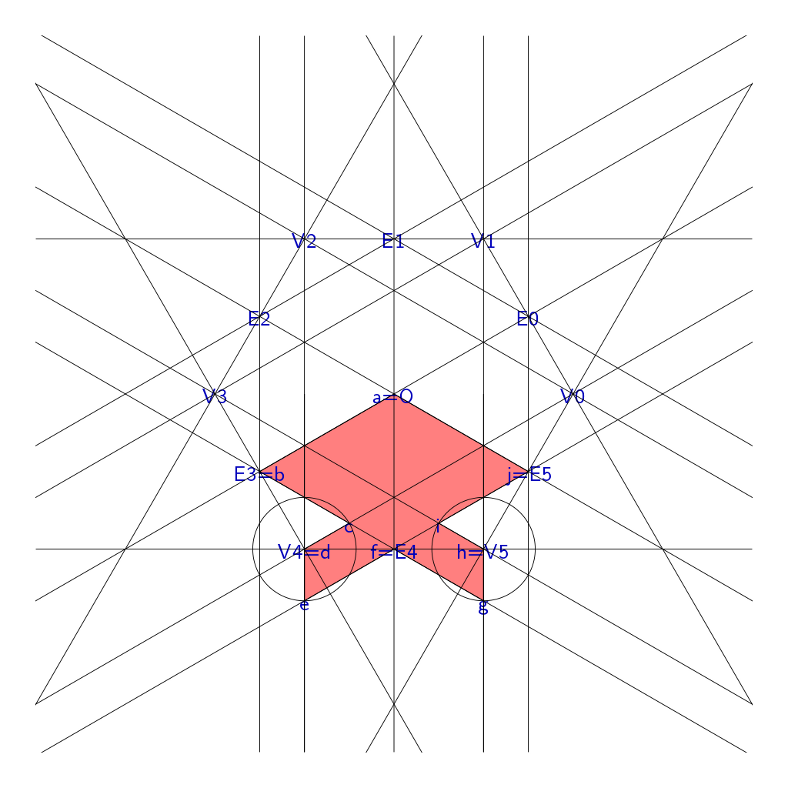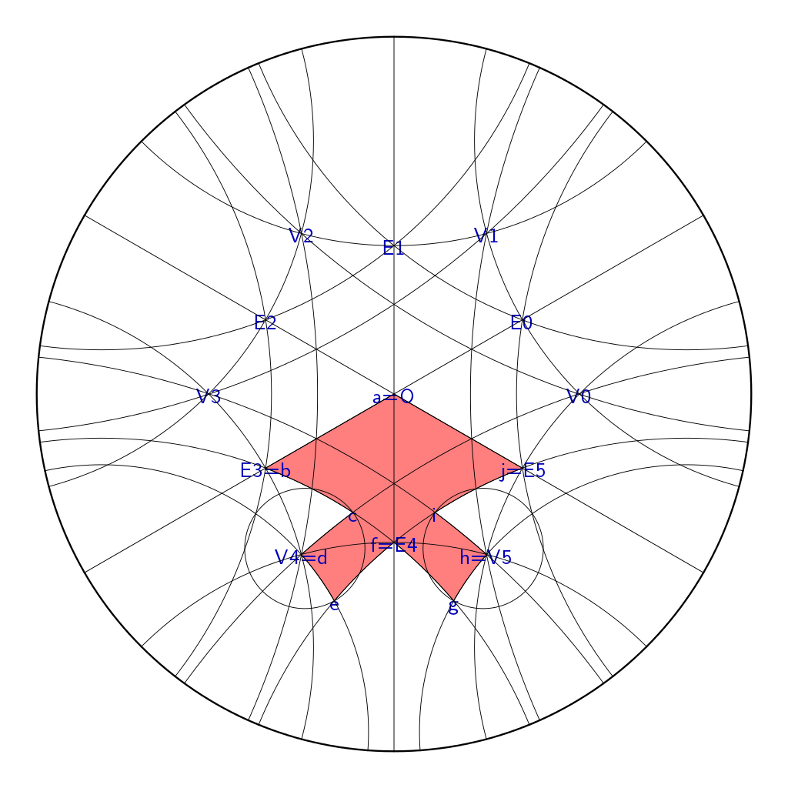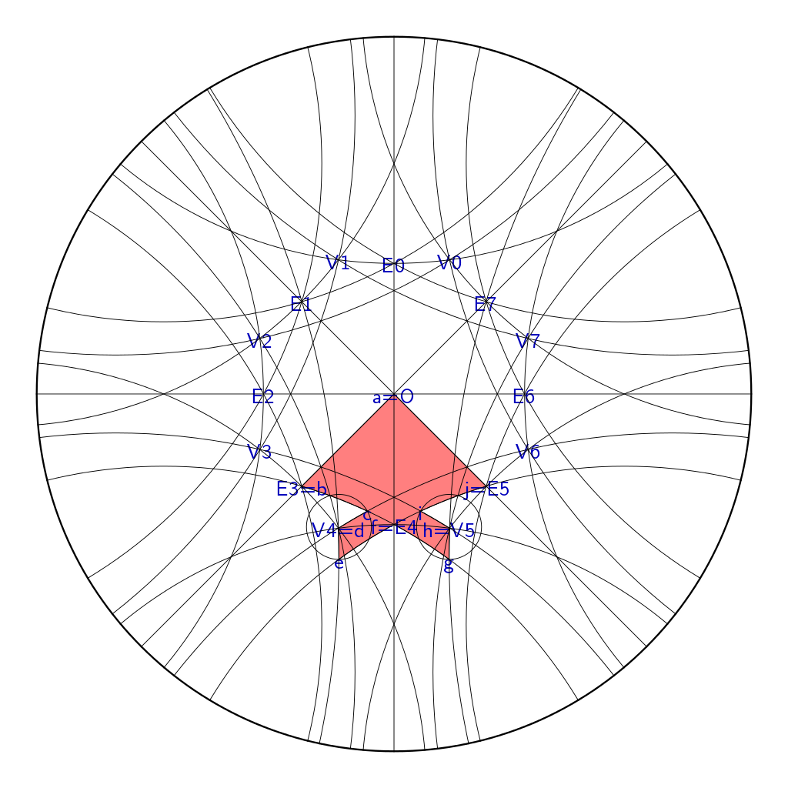# mathr / blog / #

## Fish variationsI drew part of a $$\{6,3\}$$ tiling of the plane with stylized fish, the full tiling would look more like:The shape of the fish is constructed with compass and straightedge. First create a hexagon with origin $$O$$, vertices $$V_i$$ and edge midpoints $$E_i$$. Define $$\operatorname{line}(p, q)$$ as the line passing through $$p$$ and $$q$$, and $$\operatorname{circle}(p, q)$$ as the circle centered at $$p$$ passing through $$q$$, the remaining points of the fish can be found:

\begin{align*} c &= \operatorname{line}(E_3, E_4) \cap \operatorname{line}(V_4, V_0) \\ i &= \operatorname{line}(E_4, E_5) \cap \operatorname{line}(V_5, V_3) \\ e &= \operatorname{circle}(V_4, c) \cap \operatorname{line}(E_4, E_5) \text{ furthest from } E_4 \\ g &= \operatorname{circle}(V_5, i) \cap \operatorname{line}(E_4, E_3) \text{ furthest from } E_4 \end{align*}It turns out that this construction is also valid in hyperbolic space, because nothing depends on the existence of unique parallels. Here's a diagram showing four hexagons about each vertex in the Poincaré disk model of hyperbolic geometry:And another showing three octagons about each vertex:In hyperbolic space areas and angles are connected. The key step in making hyperbolic tilings is finding the side lengths of the fundamental triangle, between the origin $$O$$, vertex $$V$$, and midpoint of neighbouring edge $$E$$. All the angles are known given the Schläfli symbol $$\{p,q\}$$: they are $$(\pi/p, \pi/q, \pi/2)$$. The side lengths can be calculated using the hyperbolic law of cosines:

\begin{align*} \cosh(|OV|) = \frac{\cos(\pi/2) + \cos(\pi/p) \cos(\pi/q)}{\sin(\pi/p) \sin(\pi/q)} \\ \cosh(|OE|) = \frac{\cos(\pi/q) + \cos(\pi/p) \cos(\pi/2)}{\sin(\pi/p) \sin(\pi/2)} \\ \cosh(|EV|) = \frac{\cos(\pi/p) + \cos(\pi/q) \cos(\pi/2)}{\sin(\pi/q) \sin(\pi/2)} \end{align*}

Here are some fish tiling variations in hyperbolic space, with Poincaré half-plane model representations too:

I wrote the implementation in Haskell. My code consists of a library for compass-and-straightedge construction with instances for Euclidean (flat) and hyperbolic (negatively curved) space with spherical (positively curved) space a possibility in the future, along with embeddings into Euclidean space for visualisation using the Diagrams library. The cool part is that the same code generates all the variations from a few parameters.